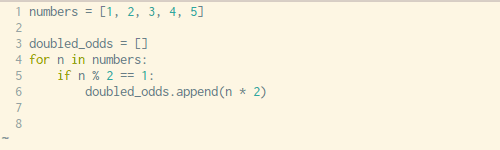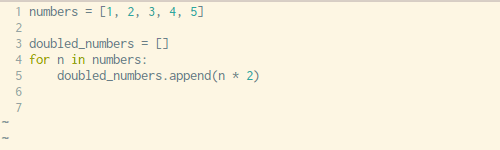# 轻松学会Python列表解析式Python中的列表解析式是个伟大的发明，但是要掌握好这个语法则有些难，因为它们并是用来解决全新的问题：只是为解决已有问题提供了新的语法。

## 什么是列表解析式？

``````>>> doubled_odds = map(lambda n: n * 2, filter(lambda n: n % 2 == 1, numbers))
>>> doubled_odds = [n * 2 for n in numbers if n % 2 == 1]

``````

## 从循环到解析式

``````:::python
new_things = []
for ITEM in old_things:
if condition_based_on(ITEM):
new_things.append("something with " + ITEM)

``````

``````:::python
new_things = ["something with " + ITEM for ITEM in old_things if condition_based_on(ITEM)]

``````

## 列表解析式：可视化解读• 将变量赋值操作复制到新建的空列表中（第三行）
• `append()`方法中的表达式参数复制到新列表中（第六行）
• 复制`for`循环语句，不包括最后的`:`（第四行）
• 复制`if`条件控制语句，同样不包括最后的`:`（第五行）

``````:::python
numbers = [1, 2, 3, 4, 5]

doubled_odds = []
for n in numbers:
if n % 2 == 1:
doubled_odds.append(n * 2)

``````

``````:::python
numbers = [1, 2, 3, 4, 5]

doubled_odds = [n * 2 for n in numbers if n % 2 == 1]

``````

## 无条件子句的列表解析式

``````:::python
doubled_numbers = []
for n in numbers:
doubled_numbers.append(n * 2)

``````

``````:::python
doubled_numbers = [n * 2 for n in numbers]

``````• 将变量赋值操作复制到新建的空列表中（第三行）
• `append()`方法中的表达式参数复制到新列表中（第五行）
• 复制`for`循环语句，不包括最后的`:`（第四行）

## 嵌套循环

``````:::python
flattened = []
for row in matrix:
for n in row:
flattened.append(n)

``````

``````:::python
flattened = [n for row in matrix for n in row]

``````

``````:::python
flattened = [n for n in row for row in matrix]

``````

## 其他解析式

``````:::python
first_letters = set()
for w in words:

``````

``````:::python
first_letters = {w for w in words}

``````

``````:::python
flipped = {}
for key, value in original.items():
flipped[value] = key

``````

``````:::python
flipped = {value: key for key, value in original.items()}

``````

## 还要注意可读性

### 列表解析式 List comprehension

``````:::python
doubled_odds = [n * 2 for n in numbers if n % 2 == 1]

``````

``````:::python
doubled_odds = [
n * 2
for n in numbers
if n % 2 == 1
]

``````

### 带嵌套循环的列表解析式

``````:::python
flattened = [n for n in row for row in matrix]

``````

``````:::python
flattened = [
n
for row in matrix
for n in row
]

``````

### 字典解析式

``````:::python
flipped = {value: key for key, value in original.items()}

``````

``````:::python
flipped = {
value: key
for key, value in original.items()
}

``````

## 总结

``````:::python
new_things = []
for ITEM in old_things:
if condition_based_on(ITEM):
new_things.append("something with " + ITEM)

``````

``````:::python
new_things = ["something with " + ITEM for ITEM in old_things if condition_based_on(ITEM)]

``````

（有删减）